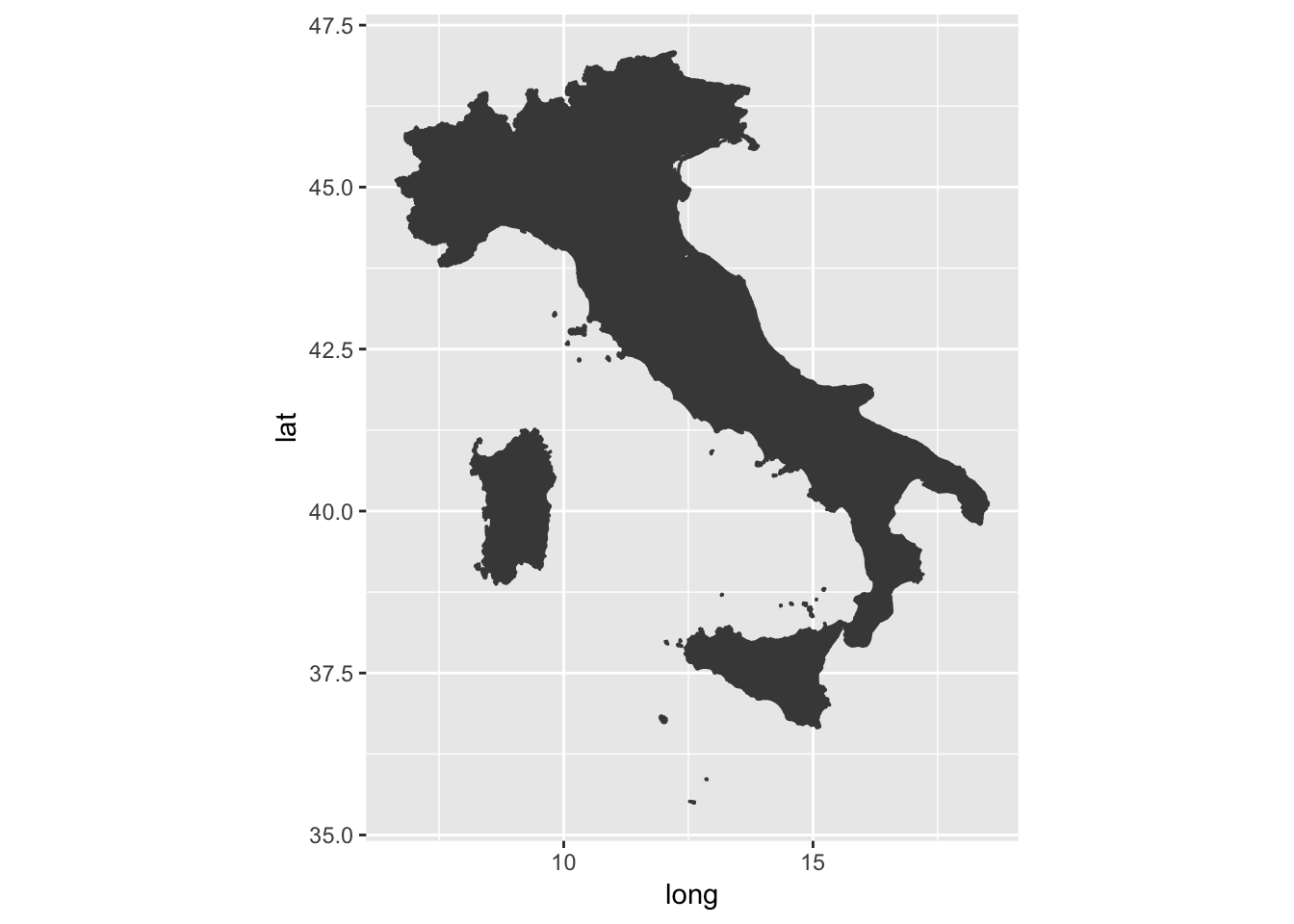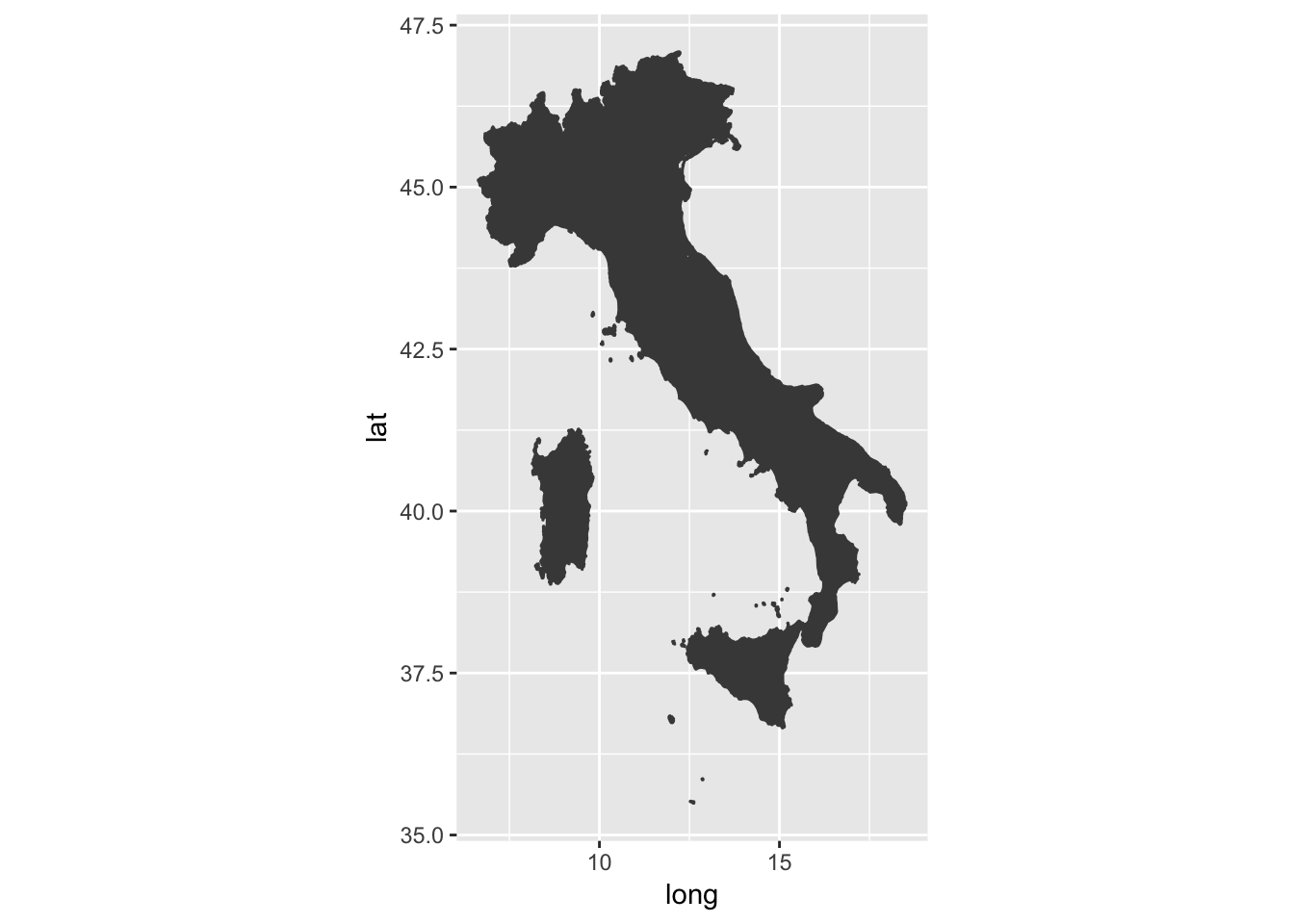``````library(ggplot2)
library(ggmap)
library(maps)
library(mapdata)
library(tidyverse)``````

## We will use the map_data function to obtain dataframes with the coordinates you need to generate maps.

``italy <- map_data("italy")``

This is what the dataframe looks like:

``head(italy)``
``````##       long      lat group order        region subregion
## 1 11.83295 46.50011     1     1 Bolzano-Bozen      <NA>
## 2 11.81089 46.52784     1     2 Bolzano-Bozen      <NA>
## 3 11.73068 46.51890     1     3 Bolzano-Bozen      <NA>
## 4 11.69115 46.52257     1     4 Bolzano-Bozen      <NA>
## 5 11.65041 46.50721     1     5 Bolzano-Bozen      <NA>
## 6 11.63282 46.48045     1     6 Bolzano-Bozen      <NA>``````

We use geom_polygon to create the different shapes. You use the fixed coordinates function to fix the aspect ratio.

``````ggplot() + geom_polygon(data = italy,
aes(x=long, y = lat, group = group),
color = "gray28", fill="gray28") +
coord_fixed(1.3)``````This looks bad with this ratio

``````ggplot() + geom_polygon(data = italy,
aes(x=long, y = lat, group = group),
color = "gray28", fill="gray28") +
coord_fixed(1.8)``````In the above, I forced the color so that you couldn’t tell the regions apart, you can change this.

``````ggplot() + geom_polygon(data = italy,
aes(x=long, y = lat, group = group)) +
coord_fixed(1.3)``````